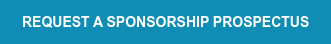<img height="1" width="1" style="display:none" alt="" src="https://www.facebook.com/tr?id=367542720414923&amp;ev=PageView&amp;noscript=1">9:30am - 1:30pm AEDT
11:30pm - 3:30pm NZDTtrue true true true true true true true true true true true true true true true true true true true true true true true true true true true true true true true true true true true true true true true true true true true true true true true true true true true true true true true true true true true true true true true true true true true true true true true true true true true true true true true true true true true true true true true true true true true true true true true true true true true true true true true true true true true true true true true true true true true true true true true true true true true true true true true true true true true true true true true true true true true true true true true true true true true true true true true true true true true true true true true true true true true true true true true true true true true true true true true true true true true true true true true true true true true true true true true true true true true true true true true true true true true true true true true true true true true true true true true true true true true true true true true true true true true true true true true